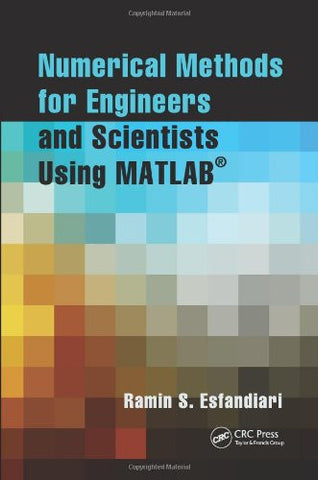Shopping Cart### Numerical Methods for Engineers and Scientists Using MATLAB®

Regular price \$136.48 Sale price

Compare at \$167.43

• ISBN-13: 9781466585690
• Publisher: CRC Press
• Release Date: Jun 04, 2013
• Edition: 1
• Pages: 550 pages
• Dimensions: 6.0 x 9.0 x 1.0 inches

### Overview

Designed to benefit scientific and engineering applications, Numerical Methods for Engineers and Scientists Using MATLAB® focuses on the fundamentals of numerical methods while making use of MATLAB software. The book introduces MATLAB early on and incorporates it throughout the chapters to perform symbolic, graphical, and numerical tasks. The text covers a variety of methods from curve fitting to solving ordinary and partial differential equations.

• Provides fully worked-out examples showing all details
• Confirms results through the execution of the user-defined function or the script file
• Executes built-in functions for re-confirmation, when available
• Generates plots regularly to shed light on the soundness and significance of the numerical results

Created to be user-friendly and easily understandable, Numerical Methods for Engineers and Scientists Using MATLAB® provides background material and a broad introduction to the essentials of MATLAB, specifically its use with numerical methods. Building on this foundation, it introduces techniques for solving equations and focuses on curve fitting and interpolation techniques. It addresses numerical differentiation and integration methods, presents numerical methods for solving initial-value and boundary-value problems, and discusses the matrix eigenvalue problem, which entails numerical methods to approximate a few or all eigenvalues of a matrix. The book then deals with the numerical solution of partial differential equations, specifically those that frequently arise in engineering and science.

The book presents a user-defined function or a MATLAB script file for each method, followed by at least one fully worked-out example. When available, MATLAB built-in functions are executed for confirmation of the results. A large set of exercises of varying levels of difficulty appears at the end of each chapter. The concise approach with strong, up-to-date MATLAB integration provided by this book affords readers a thorough knowledge of the fundamentals of numerical methods utilized in various disciplines.